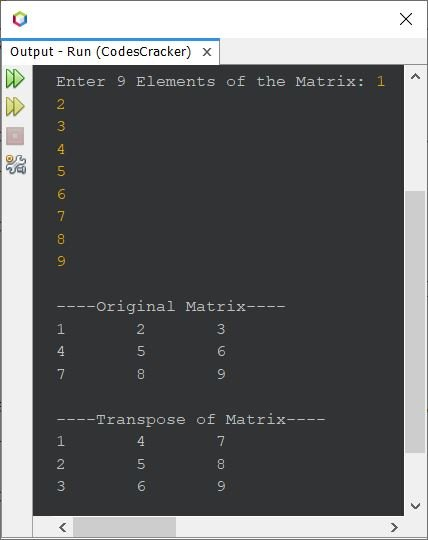# Java Program to Find the Transpose of a Matrix

This article covers a program in Java that find and prints the transpose of a given matrix. The program is created with and without using second matrix.

The transpose of a matrix can be calculated by interchanging the row elements with column elements or column elements with row elements. For example, transpose of following matrix:

```1   2
3   4```

will be:

```1   3
2   4```

## Find Transpose of a Matrix in Java

The question is, write a Java program to find the transpose of a matrix. Elements of the matrix must be received by user at run-time of the program. The program given below is its answer:

```import java.util.Scanner;

public class CodesCracker
{
public static void main(String[] args)
{
int i, j;
int[][] matOrig = new int;
int[][] matTran = new int;
Scanner scan = new Scanner(System.in);

System.out.print("Enter 9 Elements of the Matrix: ");
for(i=0; i<3; i++)
{
for(j=0; j<3; j++)
{
matOrig[i][j] = scan.nextInt();
}
}

System.out.println("\n----Original Matrix----");
for(i=0; i<3; i++)
{
for(j=0; j<3; j++)
{
System.out.print(matOrig[i][j]+ "\t");
}
System.out.print("\n");
}

// copying the transpose of matOrig to matTran
for(i=0; i<3; i++)
{
for(j=0; j<3; j++)
{
matTran[j][i] = matOrig[i][j];
}
}

System.out.println("\n----Transpose of Matrix----");
for(i=0; i<3; i++)
{
for(j=0; j<3; j++)
{
System.out.print(matTran[i][j]+ "\t");
}
System.out.print("\n");
}
}
}```

The snapshot given below shows the sample run of above program with user input 1, 2, 3, 4, 5, 6, 7, 8, 9 as nine elements for the matrix:## Transpose of a Matrix in Java without using Second Matrix

This program is similar to previous, but created in a way to transpose the matrix without using second matrix:

```import java.util.Scanner;

public class CodesCracker
{
public static void main(String[] args)
{
int i, j, x;
int[][] matrix = new int;
Scanner scan = new Scanner(System.in);

System.out.print("Enter 9 Elements of the Matrix: ");
for(i=0; i<3; i++)
{
for(j=0; j<3; j++)
matrix[i][j] = scan.nextInt();
}

System.out.println("\n----Original Matrix----");
for(i=0; i<3; i++)
{
for(j=0; j<3; j++)
System.out.print(matrix[i][j]+ "\t");
System.out.println();
}

// finding the transpose of given matrix
for(i=0; i<3; i++)
{
for(j=0; j<i; j++)
{
x = matrix[i][j];
matrix[i][j] = matrix[j][i];
matrix[j][i] = x;
}
}

System.out.println("\n----Transpose of Matrix----");
for(i=0; i<3; i++)
{
for(j=0; j<3; j++)
System.out.print(matrix[i][j]+ "\t");
System.out.println();
}
}
}```

#### Same Program in Other Languages

Java Online Test

« Previous Program Next Program »